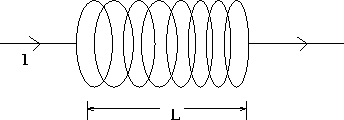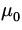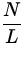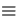## Solenoid

An extension of the previous case of a single current loop is to consider a large number N of such loops tightly packed together over a distance L - such a device is called a solenoid. This is illustrated in Fig. 1.8.This device has many important applications for two reasons: one is that fairly strong magnetic fields can be produced by using a large number of turns, and secondly, the magnetic field is fairly constant in magnitude and direction throughout the solenoid, except near the ends. The magnitude of the magnetic field of a solenoid is given by

 B =InI, (9)

where n = N/L is the number of turns per unit length. The direction of the magnetic field is given by the same rule for determining the direction of a magnetic field of a single current loop.